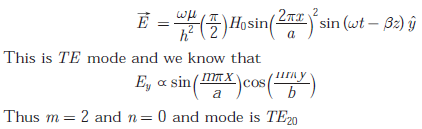# Communications - Online Test

Q1. If R(τ) is the auto correlation function of a real, wide-sense stationary random process, then which of the following is NOT true
Explaination / Solution:
No Explaination.

Q2. If S(f) is the power spectral density of a real, wide-sense stationary random process, then which of the following is ALWAYS true?
Explaination / Solution:
No Explaination.

Q3. In delta modulation, the slope overload distortion can be reduced by
Explaination / Solution:
No Explaination.

Q4. The raised cosine pulse p(t) is used for zero ISI in digital communications. The expression for p(t) with unity roll-off factor is given byThe value of p(t) at t = 1/W is
Explaination / Solution: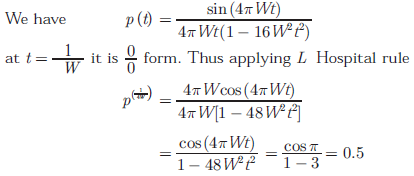Q5.
In the following scheme, if the spectrum M(f) of m(t) is as shown, then the spectrum Y(f) of y(t) will be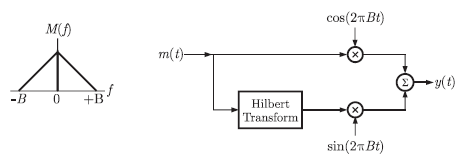Explaination / Solution: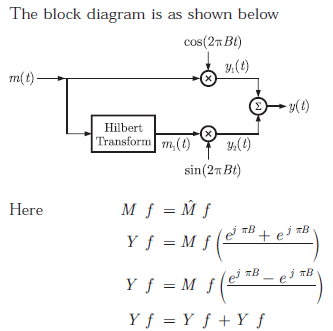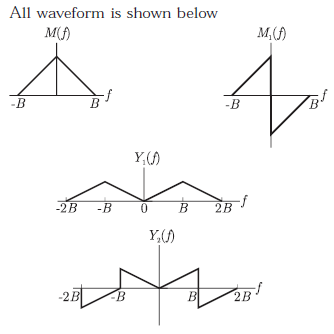Q6. During transmission over a certain binary communication channel, bit errors occur independently with probability p. The probability of AT MOST one bit in error in a block of n bits is given by
Explaination / Solution: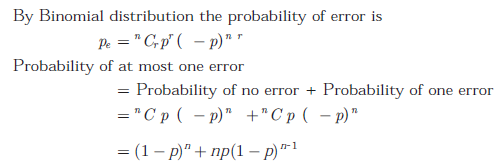Q7. In a GSM system, 8 channels can co-exist in 200 kHz bandwidth using TDMA. A GSM based cellular operator is allocated 5 MHz bandwidth. Assuming a frequency reuse factor of 1/5, i.e. a five-cell repeat pattern, the maximum number of simultaneous channels that can exist in one cell is
Explaination / Solution: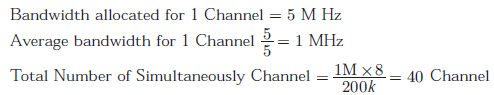Q8. In a Direct Sequence CDMA system the chip rate is 1.2288 × 106 chips per second. If the processing gain is desired to be AT LEAST 100, the data rate
Explaination / Solution:Q9. An air-filled rectangular waveguide has inner dimensions of 3 cm × 2 cm. The wave impedance of the TEmode of propagation in the waveguide at a frequency of 30 GHz is (free space impedance η0 = 377Ω)
Explaination / Solution: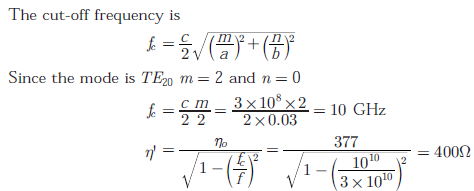Q10. The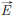field in a rectangular waveguide of inner dimension a × b is given byWhere H0 is a constant, and a and b are the dimensions along the x − axis and the y − axis respectively. The mode of propagation in the waveguide is
Explaination / Solution: